.

# Field Calculator¶

The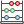Field Calculator button in the attribute table allows you to perform calculations on the basis of existing attribute values or defined functions, for instance, to calculate length or area of geometry features. The results can be written to a new attribute column, or they can be used to update values in an existing column.

You will need to bring the vector layer into editing mode, before you can click on the field calculator icon to open the dialog (see figure_attributes_3). In the dialog, you first must select whether you want to only update selected features, create a new attribute field where the results of the calculation will be added or update an existing field.

Figure Attributes 3: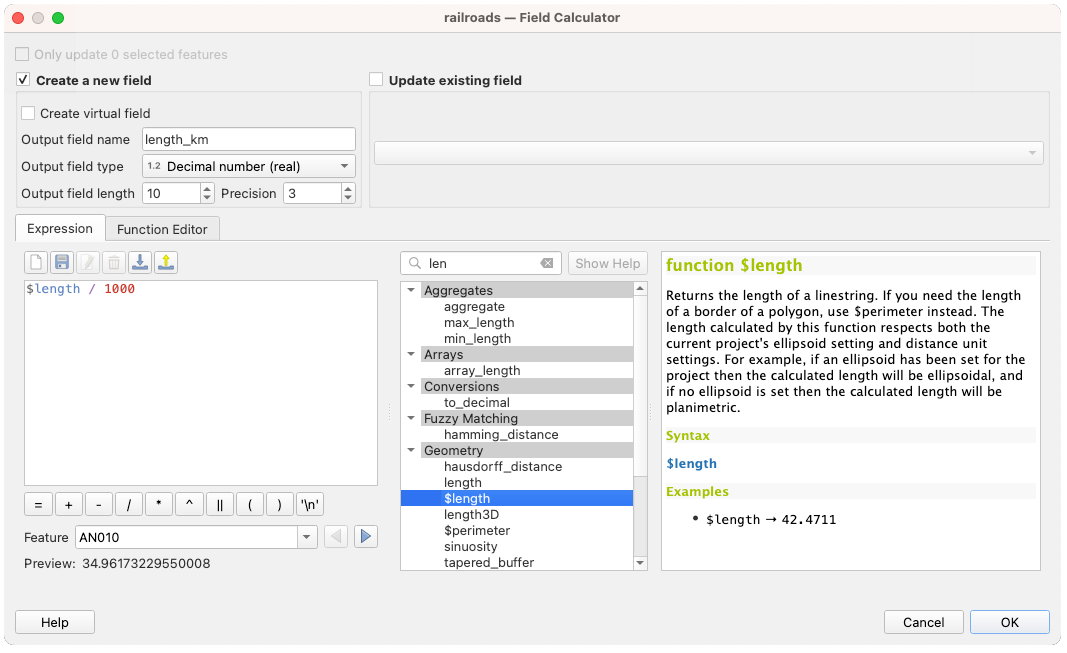Field Calculator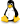If you choose to add a new field, you need to enter a field name, a field type (integer, real or string), the total field width, and the field precision (see figure_attributes_3). For example, if you choose a field width of 10 and a field precision of 3, it means you have 6 digits before the dot, then the dot and another 3 digits for the precision.

The Function List contains functions as well as fields and values. View the help function in the Selected Function Help. In Expression you see the calculation expressions you create with the Function List. For the most commonly used operators, see Operators.

In the Function List, click on Fields and Values to view all attributes of the attribute table to be searched. To add an attribute to the Field calculator Expression field, double click its name in the Fields and Values list. Generally, you can use the various fields, values and functions to construct the calculation expression, or you can just type it into the box. To display the values of a field, you just right click on the appropriate field. You can choose between Load top 10 unique values and Load all unique values. On the right side, the Field Values list opens with the unique values. To add a value to the Field calculator Expression box, double click its name in the Field Values list.

The Operators, Math, Conversions, String, Geometry and Record groups provide several functions. In Operators, you find mathematical operators. Look in Math for mathematical functions. The Conversions group contains functions that convert one data type to another. The String group provides functions for data strings. In the Geometry group, you find functions for geometry objects. With Record group functions, you can add a numeration to your data set. To add a function to the Field calculator Expression box, click on the > and then double click the function.

A short example illustrates how the field calculator works. We want to calculate the length in km of the railroads layer from the QGIS sample dataset:

1. Load the shapefile railroads.shp in QGIS and press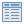Open Attribute Table.
2. Click on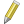Toggle editing mode and open theField Calculator dialog.
3. Select theCreate a new field checkbox to save the calculations into a new field.
4. Add length as Output field name and real as Output field type, and define Output field width to be 10 and Precision, 3.
5. Now double click on function \$length in the Geometry group to add it into the Field calculator expression box.
6. Complete the expression by typing ‘’/ 1000’’ in the Field calculator expression box and click [Ok].
7. You can now find a new column length in the attribute table.

The available functions are listed below.

The field calculator Function list with the Selected Function Help , Operators and Expression menu are also available through the rule-based rendering in the Style menu of the Layer properties, and the expression-based labelingin theLabeling core application.

Operators

This group contains operators (e.g., +, -, *).

```a + b      a plus b
a - b      a minus b
a * b      a multiplied by b
a / b      a divided by b
a % b      a modulo b (for example, 7 % 2 = 1, or 2 fits into 7 three times with remainder 1)
a ^ b      a power b (for example, 2^2=4 or 2^3=8)
a = b      a and b are equal
a > b      a is larger than b
a < b      a is smaller than b
a <> b     a and b are not equal
a != b     a and b are not equal
a <= b     a is less than or equal to b
a >= b     a is larger than or equal to b
a ~ b      a matches the regular expression b
+ a        positive sign
- a        negative value of a
||         joins two values together into a string 'Hello' || ' world'
LIKE       returns 1 if the string matches the supplied pattern
ILIKE      returns 1 if the string matches case-insensitive the supplied
pattern (ILIKE can be used instead of LIKE to make the match case-insensitive)
IS         returns 1 if a is the same as b
OR         returns 1 when condition a or b is true
AND        returns 1 when condition a and b are true
NOT        returns 1 if a is not the same as b
column name "column name"     value of the field column name
'string'                      a string value
NULL                          null value
a IS NULL                     a has no value
a IS NOT NULL                 a has a value
a IN (value[,value])          a is below the values listed
a NOT IN (value[,value])      a is not below the values listed```

Conditionals

This group contains functions to handle conditional checks in expressions.

```CASE                          evaluates multiple expressions and returns a result
CASE ELSE                     evaluates multiple expressions and returns a result
coalesce                      returns the first non-NULL value from the expression list
regexp_match                  returns true if any part of a string matches
the supplied regular expression```

Mathematical Functions

This group contains math functions (e.g., square root, sin and cos).

```sqrt(a)                       square root of a
abs                           returns the absolute value of a number
sin(a)                        sine of a
cos(a)                        cosine of a
tan(a)                        tangent of a
asin(a)                       arcsin of a
acos(a)                       arccos of a
atan(a)                       arctan of a
atan2(y,x)                    arctan of y/x using the signs of the two arguments
to determine the quadrant of the result
exp                           exponential of a value
ln                            value of the natural logarithm of the passed expression
log10                         value of the base 10 logarithm of the passed expression
log                           value of the logarithm of the passed value and base
round                         round to number of decimal places
rand                          random integer within the range specified by the minimum
and maximum argument (inclusive)
randf                         random float within the range specified by the minimum
and maximum argument (inclusive)
max                           largest value in a set of values
min                           smallest value in a set of values
clamp                         restricts an input value to a specified range
scale_linear                  transforms a given value from an input domain to an output
range using linear interpolation
scale_exp                     transforms a given value from an input domain to an output
range using an exponential curve
floor                         rounds a number downwards
ceil                          rounds a number upwards
\$pi                           pi as value for calculations```

Conversions

This group contains functions to convert one data type to another (e.g., string to integer, integer to string).

```toint                        converts a string to integer number
toreal                       converts a string to real number
tostring                     converts number to string
todatetime                   converts a string into Qt data time type
todate                       converts a string into Qt data type
totime                       converts a string into Qt time type
tointerval                   converts a string to an interval type (can be used to take days,
hours, months, etc. off a date)```

Date and Time Functions

This group contains functions for handling date and time data.

```\$now       current date and time
age        difference between two dates
year       extract the year part from a date, or the number of years from an interval
month      extract the month part from a date, or the number of months from an interval
week       extract the week number from a date, or the number of weeks from an interval
day        extract the day from a date, or the number of days from an interval
hour       extract the hour from a datetime or time, or the number
of hours from an interval
minute     extract the minute from a datetime or time, or the number
of minutes from an interval
second     extract the second from a datetime or time, or the number
of minutes from an interval```

String Functions

This group contains functions that operate on strings (e.g., that replace, convert to upper case).

```lower         convert string a to lower case
upper         convert string a to upper case
title         converts all words of a string to title case (all words lower case
with leading capital letter)
trim          removes all leading and trailing white space (spaces, tabs, etc.) from a string
length        length of string a
replace       returns a string with the supplied string replaced
regexp_replace(a,this,that)  returns a string with the supplied regular expression replaced
regexp_substr    returns the portion of a string which matches a supplied regular expression
substr(*a*,from,len)         returns a part of a string
concat        concatenates several strings to one
strpos        returns the index of a regular expression in a string
left          returns a substring that contains the n leftmost characters of the string
right         returns a substring that contains the n rightmost characters of the string
rpad          returns a string with supplied width padded using the fill character
lpad          returns a string with supplied width padded using the fill character
format        formats a string using supplied arguments
format_number   returns a number formatted with the locale separator for thousands
(also truncates the number to the number of supplied places)
format_date   formats a date type or string into a custom string format```

Color Functions

This group contains functions for manipulating colors.

```color_rgb       returns a string representation of a color based on its red, green,
and blue components
color_rgba      returns a string representation of a color based on its red, green,
blue, and alpha (transparency) components
ramp_color      returns a string representing a color from a color ramp
color_hsl       returns a string representation of a color based on its hue,
saturation, and lightness attributes
color_hsla      returns a string representation of a color based on its hue, saturation,
lightness and alpha (transparency) attributes
color_hsv       returns a string representation of a color based on its hue,
saturation, and value attributes
color_hsva      returns a string representation of a color based on its hue, saturation,
value and alpha (transparency) attributes
color_cmyk      returns a string representation of a color based on its cyan, magenta,
yellow and black components
color_cmyka     returns a string representation of a color based on its cyan, magenta,
yellow, black and alpha (transparency) components```

Geometry Functions

This group contains functions that operate on geometry objects (e.g., length, area).

```xat              retrieves an x coordinate of the current feature
yat              retrieves a y coordinate of the current feature
\$area            returns the area size of the current feature
\$length          returns the length size of the current feature
\$perimeter       returns the perimeter length of the current feature
\$x               returns the x coordinate of the current feature
\$y               returns the y coordinate of the current feature
\$geometry        returns the geometry of the current feature (can be used
for processing with other functions)
geomFromWKT      returns a geometry created from a well-known text (WKT) representation
geomFromGML      returns a geometry from a GML representation of geometry
bbox
disjoint         returns 1 if the geometries do not share any space together
intersects       returns 1 if the geometries spatially intersect
(share any portion of space) and 0 if they don't
touches          returns 1 if the geometries have at least one point in common,
but their interiors do not intersect
crosses          returns 1 if the supplied geometries have some, but not all,
interior points in common
contains         returns true if and only if no points of b lie in the exterior of a,
and at least one point of the interior of b lies in the interior of a
overlaps         returns 1 if the geometries share space, are of the same dimension,
but are not completely contained by each other
within           returns 1 if geometry a is completely inside geometry b
buffer           returns a geometry that represents all points whose distance
from this geometry is less than or equal to distance
centroid         returns the geometric center of a geometry
convexHull       returns the convex hull of a geometry (this represents the
minimum convex geometry that encloses all geometries within the set)
difference       returns a geometry that represents that part of geometry a that
does not intersect with geometry b
distance         returns the minimum distance (based on spatial ref) between
two geometries in projected units
intersection     returns a geometry that represents the shared portion
of geometry a and geometry b
symDifference    returns a geometry that represents the portions of a and b
that do not intersect
combine          returns the combination of geometry a and geometry b
union            returns a geometry that represents the point set union of the geometries
geomToWKT        returns the well-known text (WKT) representation of the
geometry without SRID metadata```

Record Functions

This group contains functions that operate on record identifiers.

```\$rownum                      returns the number of the current row
\$id                          returns the feature id of the current row
\$scale                       returns the current scale of the map canvas```

Fields and Values

Contains a list of fields from the layer. Sample values can also be accessed via right-click.

Select the field name from the list, then right-click to access a context menu with options to load sample values from the selected field.